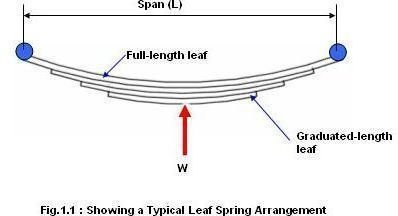# Leaf Spring Design Guidelines – Laminated Leaf Spring Design Procedures, Formula and Example

A leaf spring protects a four wheeler from the unevenness of the road. Thus a leaf spring necessarily serves the following purposes:

• Increase service life of a four wheeler
• Increase user comfort

## What an Automobile Leaf Spring Looks LikeAs shown in the picture, the leaf spring or laminated leaf spring consists of a couple of full-length leaf and a number of graduated leaves. You can also observe from the picture of the leaf spring that the thickness of the whole spring is maximum at the centre and is gradually decreases towards the ends.

## Formula to be used

Leaf spring design is based on the fundamental beam theory. I already have an article on how to design plates based on beam theory.

You have to use the following two formulas for the leaf spring design process:

• Bending stress produced in the whole spring:

Tb= (3*W*L)/ (b*N*t^2)………………………………eqn1.1

• Maximum deflection in the whole spring:

X= (6*W*L^3)/[{(2+(n/N)}*E*N*b*t^3]……………eqn1.2

Where,

Tb – maximum bending stress in the leaf spring

W – Applied load on the spring

t – Thickness of the individual leaves

X – Maximum deflection of the spring due to applied load

L – Span of the leaf spring

n – Number of full-length leaves

N – Number of graduated-length leaves

b – Width of the spring

E – Young’s modulus of the spring material

## Leaf Spring Design Procedure

Let’s start with a leaf spring design problem. We have the following data as input:

W=24516N

L=0.445M

N=8

n=2

E=2.1E+11N/m2

X=0.13M

Sa=600000000N/m2

Editor’s Note: A reader has pointed out that the correct formula may be E = 2.1 x 10^11 N/m^2 rather than the stated E = 2.1 E + 11 N/m^2

Sa is the allowable bending stress for the spring material.

You have to find out the width (b) and the thickness (t) of the spring.

Solution:

Apply the eqn1.1 and you will get the value of:

b*t^2 = 6.81 * 10^-6…………eqn1.3

Now, apply the eqn1.2 and you will get the value of:

b*t^3 = 2.63 * 10^-8………….eqn1.4

By solving the eqn1.3 and eqn1.4 you will get:

t = 0.0038 m

b = 0.455 m

## Conclusion

User comfort and the service life of a four wheeler is dependent on the design of leaf spring. Leaf spring design guidelines explained here is the simplest version of the whole leaf spring design procedure. Nowadays, CAD and FEA tools are used extensively in the industry for the design of leaf spring.